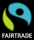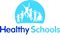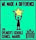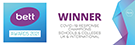# Being a Mathematician

Our mathematicians are provided with a foundation for understanding the world, the ability to reason mathematically, an appreciation of the beauty and power of mathematics, and a sense of enjoyment and curiosity about the subject.

At St Barnabas, teachers follow the White Rose Mastery Scheme of Work and use manipulatives and pictures to support their understanding. In Reception, children are mathematicians as part of their play provision. Children are given opportunities to explore all different aspects of being a mathematician and to use and apply reasoning skills. Activities are either adult-led, adult initiated or child-initiated.

Through Key Stage 1, our mathematicians begin to record their work in more formal calculations. They learn about addition, subtraction, sharing and grouping. By Year 2, our mathematicians are taught multiplication and division and will learn the 2, 3, 5 and 10 times tables. They will begin to tell the time using an analogue clock, use money, describe simple 2D and 3D shapes and read scales for measuring length, height and quantity. In Key Stage 2, our mathematicians build on this knowledge and learn more times tables (2, 3, 4, 5, 6, 7, 8, 9, 11 and 12). They learn to use this knowledge and all the other number facts they have learnt so far to apply to more complex calculations and problem solving. They learn a range of more complex methods for solving calculations with 2, 3 and 4-digit numbers including decimals. Our mathematicians are taught about fractions, percentages and decimal places. They will learn the mathematical skills essential for life!